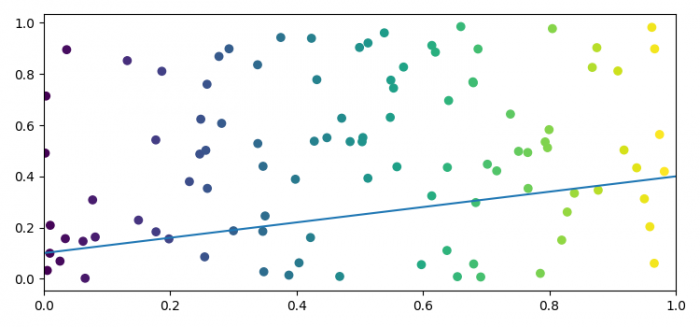# Adding a line to a scatter plot using Python's Matplotlib

To add a line to a scatter plot using Python's Matplotlib, we can take the following steps −

• Set the figure size and adjust the padding between and around the subplots.
• Initialize a variable, n, for number of data points.
• Plot x and y data points using scatter() method.
• Plot a line using plot() method.
• Limt the X-axis using xlim() method.
• To display the figure, use show() method.

## Example

import numpy as np
from matplotlib import pyplot as plt

plt.rcParams["figure.figsize"] = [7.50, 3.50]
plt.rcParams["figure.autolayout"] = True

n = 100
x = np.random.rand(n)
y = np.random.rand(n)

plt.scatter(x, y, c=x)
plt.plot([0.1, 0.4, 0.3, 0.2])
plt.xlim(0, 1)

plt.show()

## Output